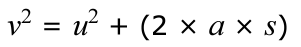# Knowledge questions for Movement and Position for Edexcel IGCSE Physics

These questions are part of the Forces and Motion Topic

The Edexcel IGCSE Physics Specification can be found here.

1. Plot a distance-time graph and explain its major features of it.
2. Write down an equation to calculate the average speed of a moving object.
3. Write down the equation to calculate the acceleration of a moving object.
4. What are the inits of speed, time, velocity and acceleration?
5. Plot and explain the main features of a velocity-time graph.
6. How can acceleration be calculated using a velocity-time graph?
7. How can distance be calculated using a velocity-time graph?
8. What do all the terms mean in this equation:-